## The Final exam

The final exam will take place August 3, 2017 at the usual time in the usual classroom. We have a global review on August 1, 2017 (the Swiss national holiday!). The average was 76/100 the standard deviation was 17 (when the exam is scaled to 100), The maximal achieved final score this year was 136.
 The exam Solution draft

A souvenir: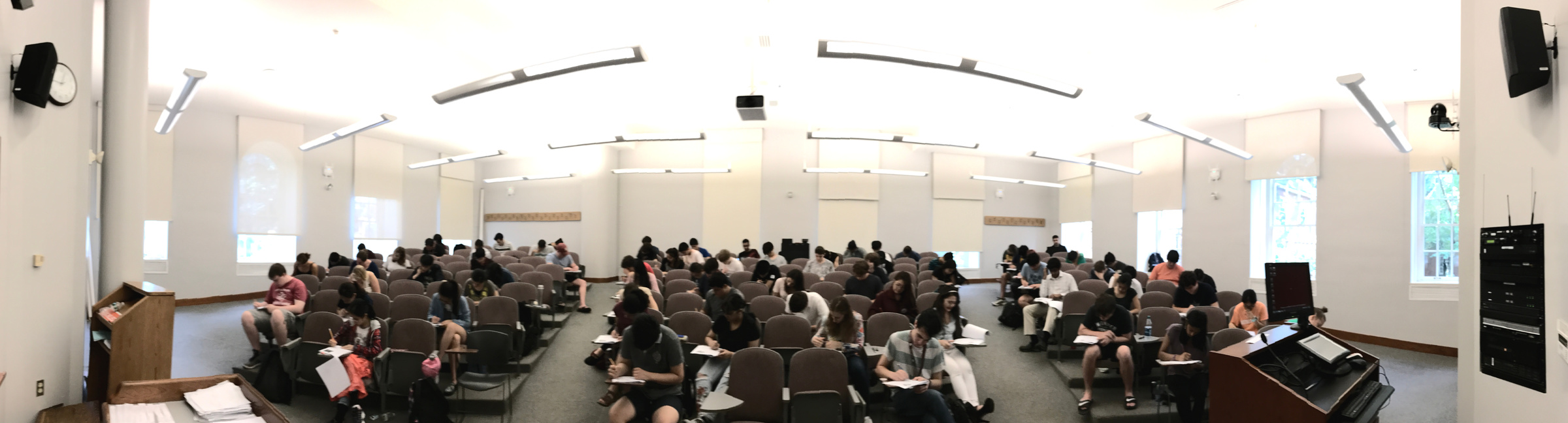Some practice exams: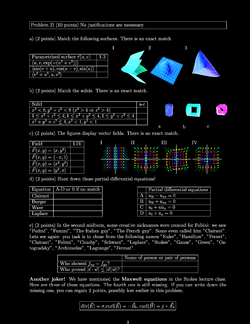Practice I, [Solutions]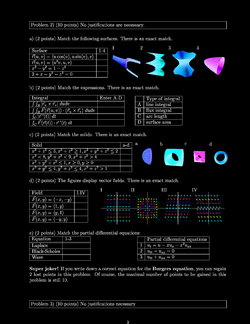Practice II, [Solutions]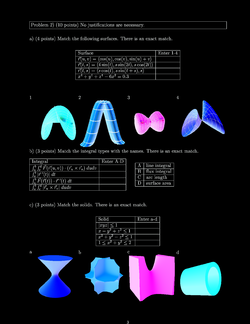Practice III, [Solutions]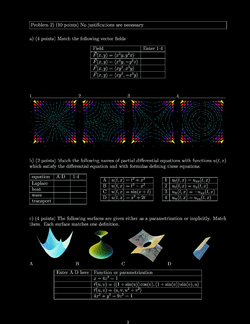Practice IV, [Solutions]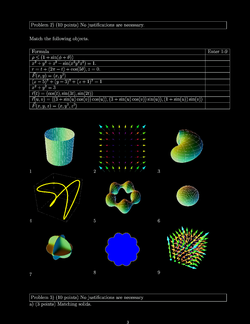Practice V, [Solutions]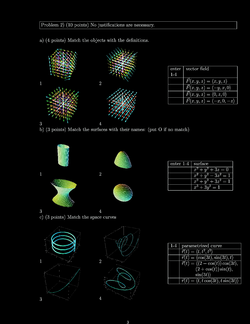Practice VI, [Solutions]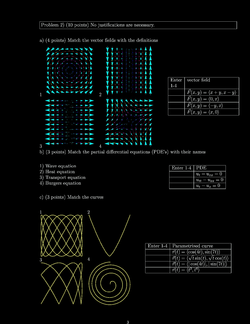Practice VII, [Solutions]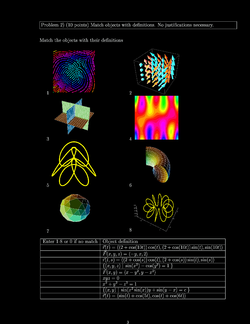Practice VIII, [Solutions]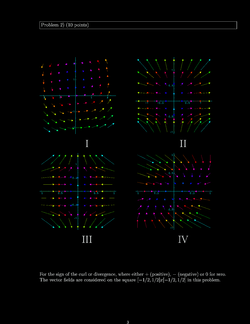Practice IV, [Solutions]

## Second hourly

The second midterm took place on July 20, 2017. It covered the material from the third and fourth week. We had our class review on Tuesday, July 18, 2017. The exam had a mean of 79 and a standard deviation of 15. Again, one student got 100/100 points. Here is the exam with solution:
 The exam Solution draft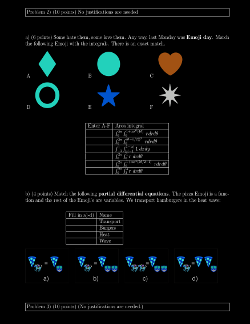Practice I, [Solutions]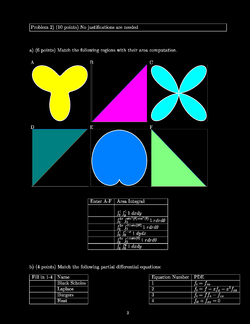Practice II, [Solutions]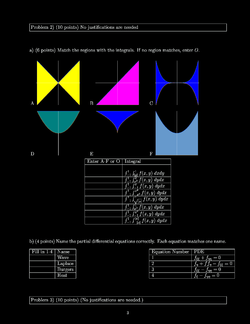Practice III, [Solutions]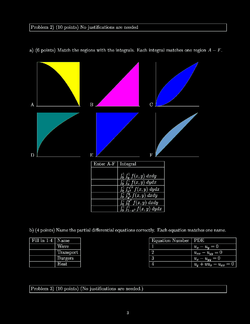Practice IV, [Solutions]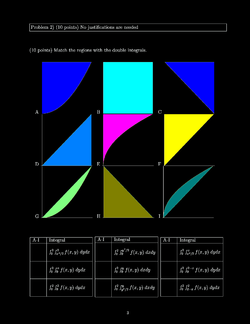Practice V, [Solutions]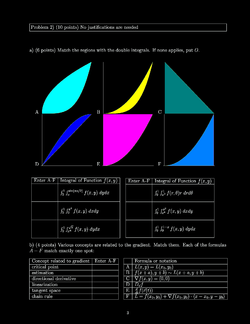Practice VI, [Solutions]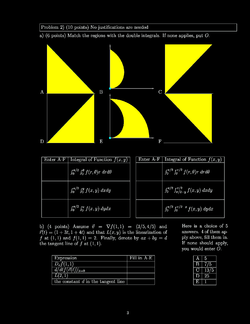Practice VII, [Solutions]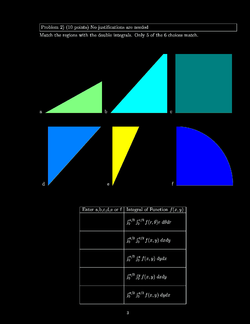Practice VIII, [Solutions]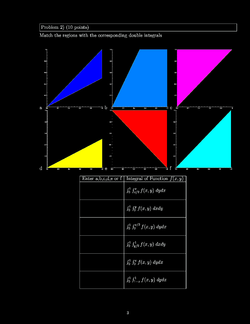Practice IX, [Solutions]

## First hourly

The first hourly took place on July 6, 2017. There was a class review on Monday, July 3 (7-9 PM in Lecture Hall C). The exam had a mean of 78 and a standard deviation of 14. One student got 100/100 points. Here is the exam with solution:
 The exam Solution draft

Here were some practice exams: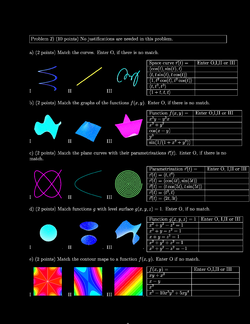Practice I, [Solutions]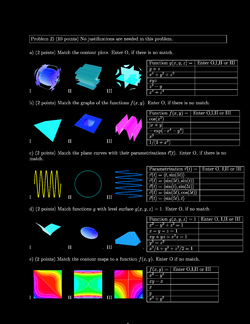Practice II, [Solutions]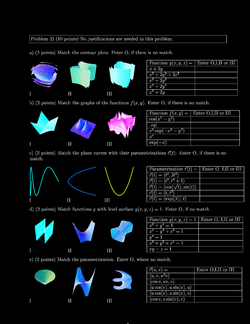Practice III, [Solutions]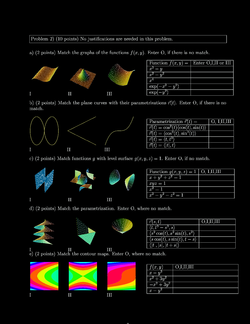Practice IV, [Solutions]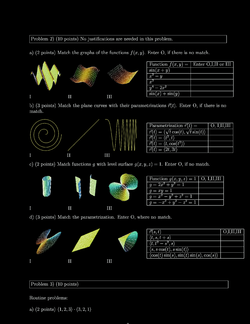Practice V, [Solutions]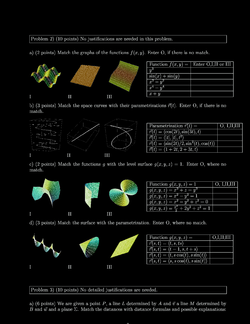Practice VI, [Solutions]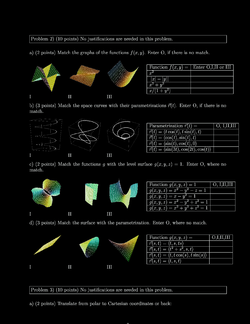Practice VII, [Solutions]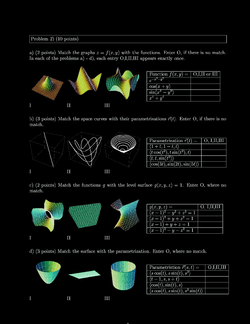Practice VIII, [Solutions]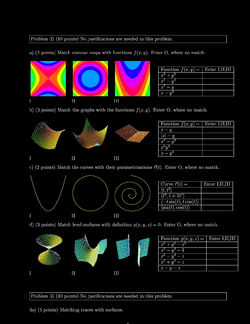Practice IX, [Solutions]

## The Final exam

The final exam takes place on August 3, 2017.